Write Numbers up to 20 Math Video for Kids - K, 1st, & 2nd Grades
1%
It was processed successfully!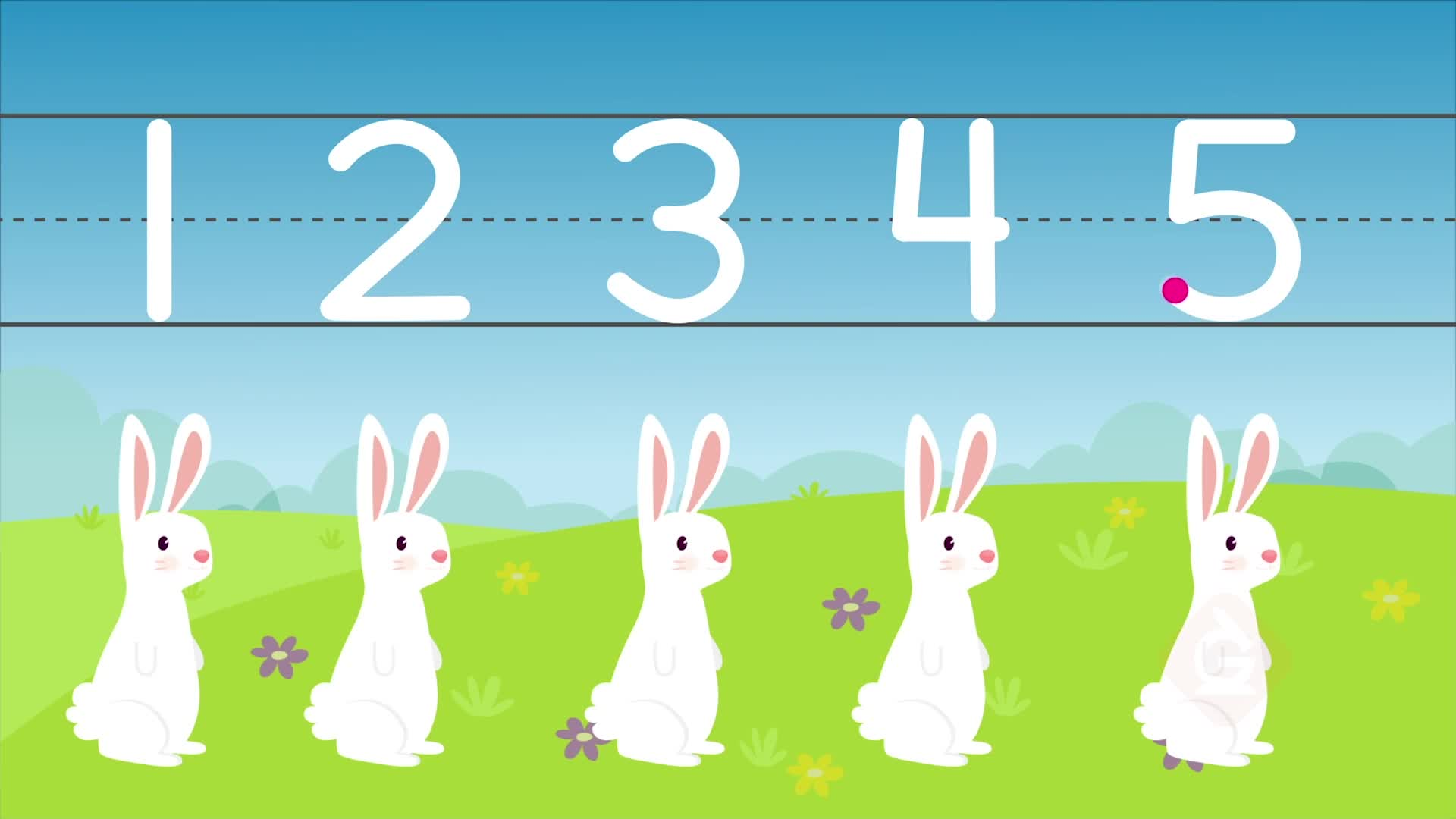HOW DO YOU WRITE NUMBERS?

You can write numbers. Pay attention to the order when you write numbers that are 10 or more.

To better understand writing numbers up to 20…

HOW DO YOU WRITE NUMBERS?. You can write numbers. Pay attention to the order when you write numbers that are 10 or more. To better understand writing numbers up to 20…

## LET’S BREAK IT DOWN!

### Count bunnies from zero to five.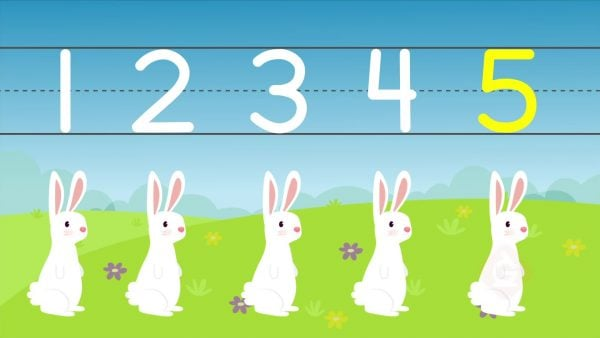There are zero bunnies: 0. Zero means you don’t have any. Here is one bunny: 1. Here are two bunnies: 2. Here are three bunnies: 3. Here are four bunnies: 4. Here are five bunnies: 5.

Count bunnies from zero to five. There are zero bunnies: 0. Zero means you don’t have any. Here is one bunny: 1. Here are two bunnies: 2. Here are three bunnies: 3. Here are four bunnies: 4. Here are five bunnies: 5.

### Count dinosaurs from six to ten.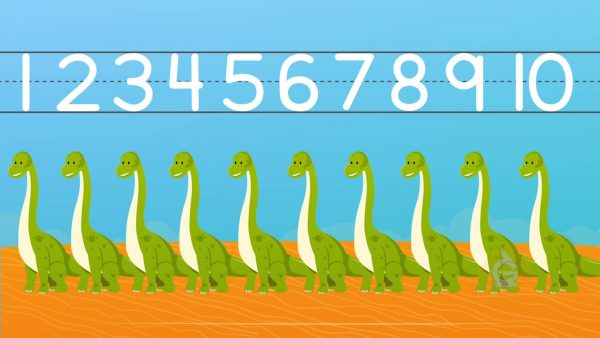There are six dinosaurs: 6. There are seven dinosaurs: 7. There are eight dinosaurs: 8. There are nine dinosaurs: 9. There are ten dinosaurs: 10.

Count dinosaurs from six to ten. There are six dinosaurs: 6. There are seven dinosaurs: 7. There are eight dinosaurs: 8. There are nine dinosaurs: 9. There are ten dinosaurs: 10.

### Count eleven robots.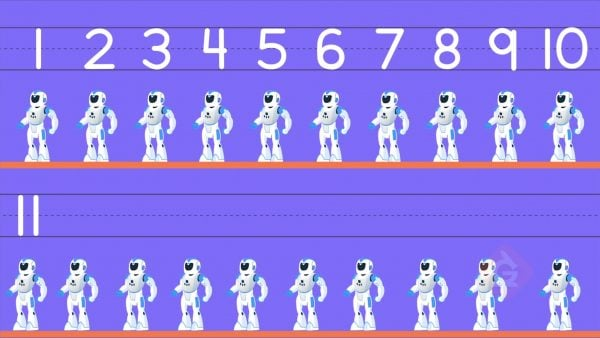There are eleven robots. To write eleven, write a 1 and then a 1 next to it: 11. 11 is the same as 1 ten and 1 one. The first number represents how many tens you have. The second number represents how many ones you have.

Count eleven robots. There are eleven robots. To write eleven, write a 1 and then a 1 next to it: 11. 11 is the same as 1 ten and 1 one. The first number represents how many tens you have. The second number represents how many ones you have.

### Count more robots.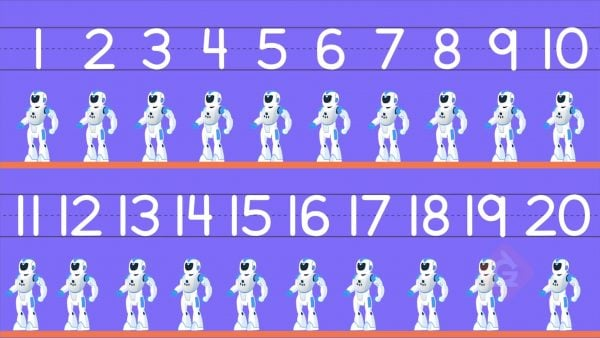There are more robots. To write twelve, write a 1 and then a 2: 12. This is 1 ten and 2 ones. To write thirteen, write a 1 and then a 3: 13. This is 1 ten and 3 ones. Keep going. 14. 15. 16. 17. 18. 19. 20. To write twenty, write 20. This is 2 tens and 0 ones.

Count more robots. There are more robots. To write twelve, write a 1 and then a 2: 12. This is 1 ten and 2 ones. To write thirteen, write a 1 and then a 3: 13. This is 1 ten and 3 ones. Keep going. 14. 15. 16. 17. 18. 19. 20. To write twenty, write 20. This is 2 tens and 0 ones.

### Count balloons.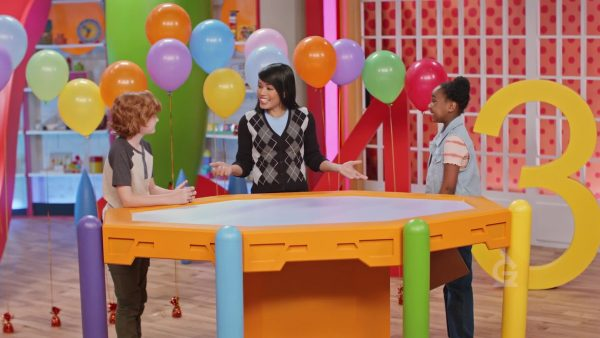There are fifteen balloons. Fifteen is 1 ten and then 5 ones, so write a 1 and then a 5: 15.

Count balloons. There are fifteen balloons. Fifteen is 1 ten and then 5 ones, so write a 1 and then a 5: 15.

## WRITE NUMBERS UP TO 20 VOCABULARY

Count
Say numbers in order to find out how many of something there are.
Numbers
Symbols used to represent how many of something there are.
Total
The result of counting.
Ten
1 ten is the same as 10 ones
Zero (0)
A zero is used to show that there are none of something. If you have no toys, you have 0 toys.
Teen Numbers
The numbers 11 to 19.

4.

10.

16.

20.

13.
X

## Success

We’ve sent you an email with instructions how to reset your password.
Ok

## Choose Your Free Trial Period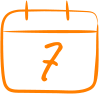### 7 DaysContinue to Lessons### 90 DaysGet 90 days free by inviting other teachers to try it too.

Share with Teachers

## Get 90 Days Free

### By inviting 4 other teachers to try it too.

4 required

Skip, I will use a 7 days free trial

## Thank You!

Enjoy your free 90 days trial